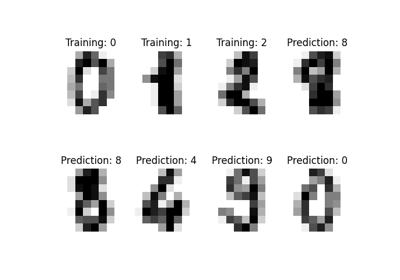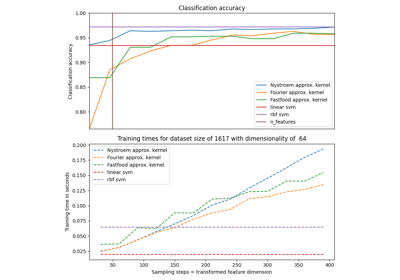sklearn_extra.kernel_approximation.Fastfood¶

Approximates feature map of an RBF kernel by Monte Carlo approximation of its Fourier transform.

Fastfood replaces the random matrix of Random Kitchen Sinks (RBFSampler) with an approximation that uses the Walsh-Hadamard transformation to gain significant speed and storage advantages. The computational complexity for mapping a single example is O(n_components log d). The space complexity is O(n_components). Hint: n_components should be a power of two. If this is not the case, the next higher number that fulfills this constraint is chosen automatically.

Parameters
sigmafloat

Parameter of RBF kernel: exp(-(1/(2*sigma^2)) * x^2)

n_componentsint

Number of Monte Carlo samples per original feature. Equals the dimensionality of the computed feature space.

mem: This version is not as accurate as the option “accuracy”,

but is consuming less memory.

accuracy: The final feature space is of dimension 2*n_components,

while being more accurate and consuming more memory.

random_state{int, RandomState}, optional

If int, random_state is the seed used by the random number generator; if RandomState instance, random_state is the random number generator.

Notes

See “Fastfood | Approximating Kernel Expansions in Loglinear Time” by Quoc Le, Tamas Sarl and Alex Smola.

Examples

See scikit-learn-fastfood/examples/plot_digits_classification_fastfood.py for an example how to use fastfood with a primal classifier in comparison to an usual rbf-kernel with a dual classifier.